# Synthetic Aperture Radar System Simulation and Image Formation

This example demonstrates how to model a Synthetic Aperture RADAR (SAR) system using stepped frequency modulated (SFM) waveform and generate a SAR image in Simulink®. In this example, a SAR platform is defined along with the waveform it transmits. The receiver is modeled to handle the matched filtering and azimuth processing of different subpulses of the SFM waveform. For a similar example in MATLAB® using linear frequency modulated (LFM) waveform, see Stripmap Synthetic Aperture Radar (SAR) Image Formation.

### Introduction

The model shows a SAR system setup to simulate IQ returns and perform image formation from the IQ data. The airborne platform in this example exploits the SFM waveform and its corresponding processing. The SFM waveform is an alternative technique to obtain a larger bandwidth, and it has some advantages over the conventional LFM waveform.

As the SFM constructs a wideband chirp from a burst of narrowband chirps, it provides improved noise figure and receiver sensitivity because of the narrow instantaneous bandwidth.

```helperslexSARSystemSim('openModel'); ```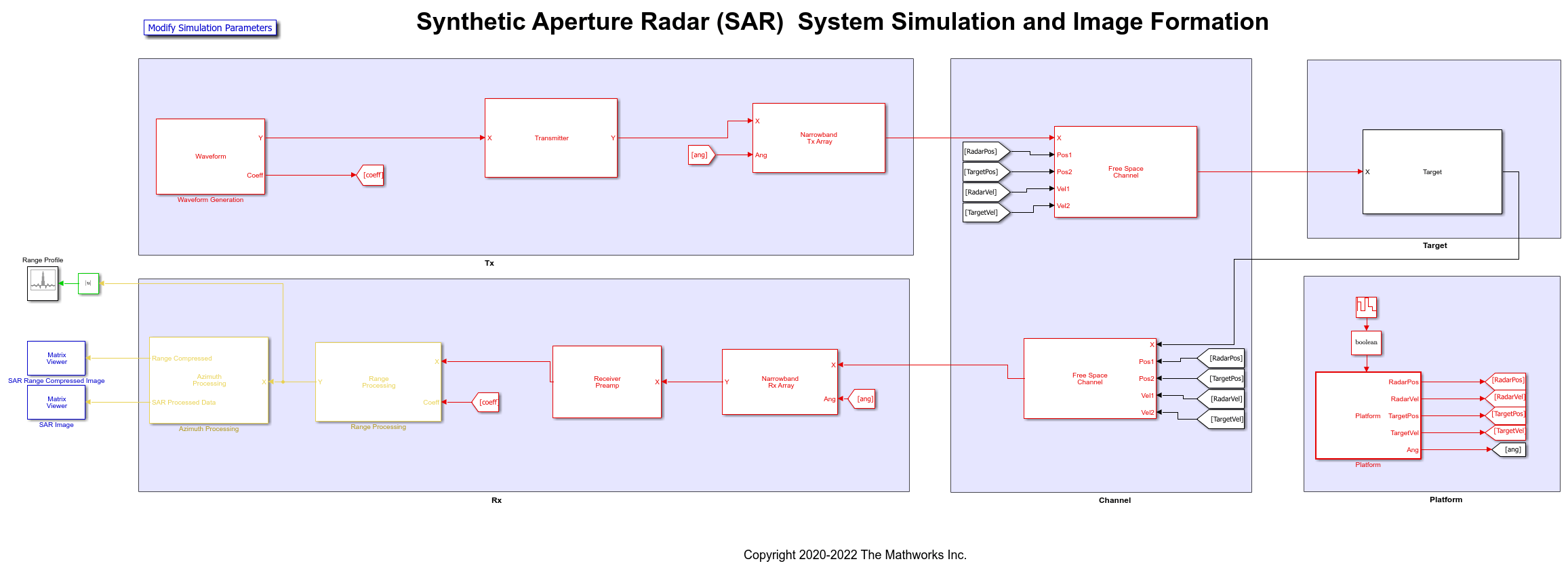The model has various sections: Tx, Channel, Target, Platform, and Rx. The Tx section simulates the generation and transmission of the SFM waveform. The Channel section models the two-way propagation of the signal to and from the target. The Target section models three point targets located at 800 m, 1000 m, and 1300 m, each with a mean RCS of 1 m^2/m^2. The Platform subsystem models the flight path. The Rx section simulates the reception, range, and azimuth processing of the SAR raw data.

Waveform Generation

The Waveform Generation subsystem includes an SFM waveform generating module. The transmitted SFM burst consists of four subpulses with the PRF of 1000 Hz, such that it satisfies the criteria for maximum unambiguous range and maximum Doppler. The coefficients for matched filtering the IQ data at the receiver are also generated in this module.

```helperslexSARSystemSim('showWaveform'); ```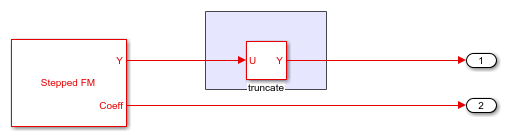Platform

The Platform subsystem simulates the motion of aircraft on which the synthetic aperture radar is mounted. It is a triggered subsystem that mimics the stop-and-go assumption as the platform moves from position A to position B, transmitting and receiving the burst of pulses at each position. Hence, the platform remains at a position until all the subpulse in a burst are transmitted and received. The subpulses here implies the four steps of the SFM waveform transmitted and received at each position along the flight path. The platform is at a height of 500 m moving in the cross-range direction at a velocity of 100 m/s.

```helperslexSARSystemSim('showPlatform'); ```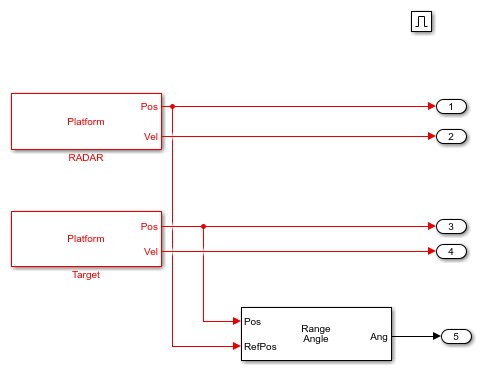Range Processing

This is the first step in obtaining the SAR image. The Range Processing subsystem depicts the matched filtering for the waveform burst. The most convenient method for matched filtering the SFM waveform burst is the pulse-by-pulse processing. In pulse-by-pulse processing, first, each individual subpulse in the burst is match filtered using the matched coefficients generated from the waveform generation subsystem. Secondly, the subpulses are integrated to complete the matched filtering of the entire burst. The pulse compressed subpulses are coherently integrated to obtain the range compressed data.

```helperslexSARSystemSim('showRangeProcessing'); ```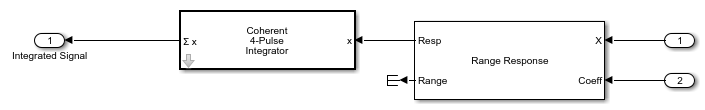Azimuth Processing

The Azimuth Processing subsystem models the final step in obtaining the SAR image. This subsystem implements range migration algorithm (RMA) to focus the SAR image. The range compressed data is buffered to obtain data from every range bin before performing azimuth processing, which focuses the image in the cross-range or azimuth direction.

```helperslexSARSystemSim('showAzimuthProcessing'); ```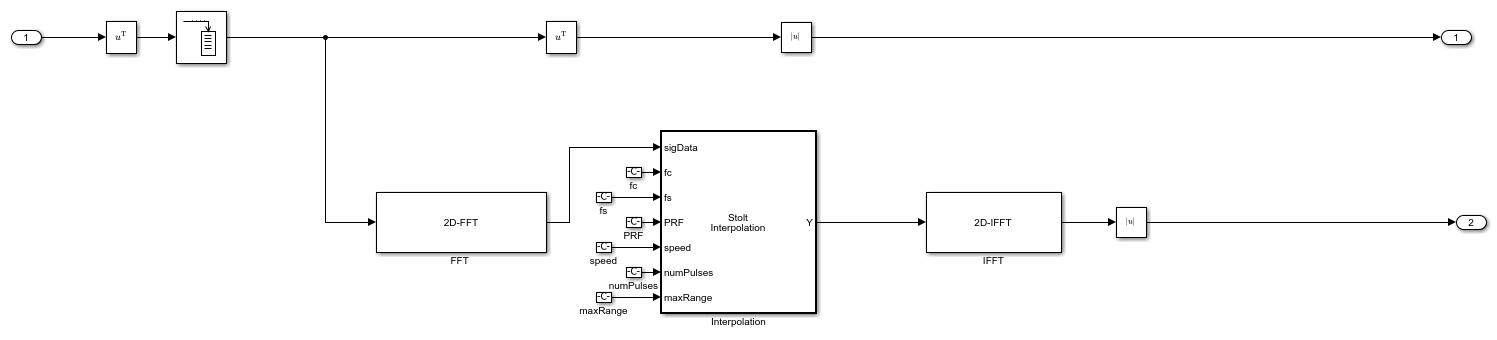### Exploring the Example

Several dialog parameters of the model are calculated by the helper function helperslexSARSystemParam. To open the function from the model, click the `Modify Simulation Parameters` block. This function is executed once the model is loaded. It exports to the workspace a structure whose fields are referenced by the dialog boxes. To modify any parameters, either change the values in the structure at the command prompt or edit the helper function and rerun it to update the parameter structure.

### Results and Display

The results show the processed data at different stages of the simulation.

```helperslexSARSystemSim('runModel'); ```

This image shows the data after range processing of the burst waveform. Run the helper function.

```helperslexSARSystemSim('showRangeCompressed'); ```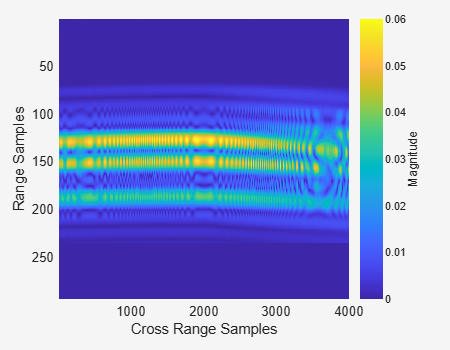This image shows the SAR image after range and azimuth focusing.

```helperslexSARSystemSim('showSARImage'); ```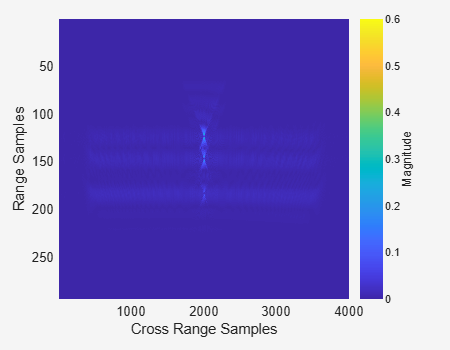### Summary

This example shows how to use an SFM waveform for a SAR imaging system mounted on an aircraft to image targets on the ground. The example models the entire system in Simulink, and shows how to use the SFM waveform and its corresponding processing.

```helperslexSARSystemSim('closeModel'); ```